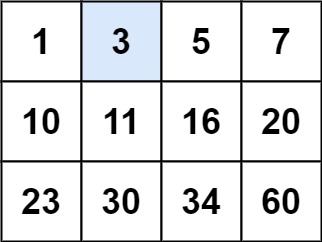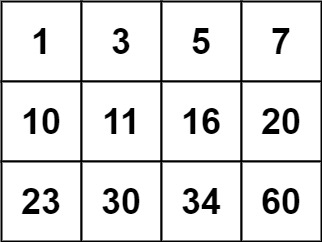# 74. Search a 2D Matrix

Medium
Write an efficient algorithm that searches for a value `target` in an `m x n` integer matrix `matrix`. This matrix has the following properties:
• Integers in each row are sorted from left to right.
• The first integer of each row is greater than the last integer of the previous row.
Example 1:Input: matrix = [[1,3,5,7],[10,11,16,20],[23,30,34,60]], target = 3
Output:
true
Example 2:Input: matrix = [[1,3,5,7],[10,11,16,20],[23,30,34,60]], target = 13
Output:
false
Constraints:
• `m == matrix.length`
• `n == matrix[i].length`
• `1 <= m, n <= 100`
• `-104 <= matrix[i][j], target <= 104`

### 解題

Runtime: 4 ms, faster than 72.55%
Memory Usage: 2.6 MB, less than 100%
func searchMatrix(matrix [][]int, target int) bool {
for _, row := range matrix {
if target > row[len(row) - 1] {
continue
} else{
return binarySearch(row, target)
}
}
return false
}
func binarySearch(row []int, target int) bool {
left, right := 0, len(row) - 1
for left <= right {
mid := (left + right) / 2
if row[mid] == target {
return true
} else if row[mid] > target {
right = mid - 1
} else {
left = mid + 1
}
}
return false
}